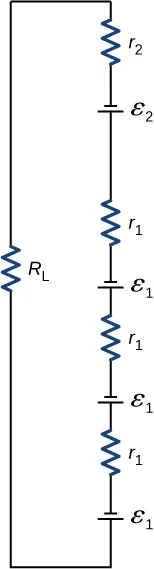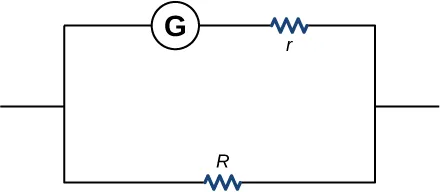University Physics Volume 2

# Chapter 10

10.1

If a wire is connected across the terminals, the load resistance is close to zero, or at least considerably less than the internal resistance of the battery. Since the internal resistance is small, the current through the circuit will be large, $I=εR+r=ε0+r=εr.I=εR+r=ε0+r=εr.$ The large current causes a high power to be dissipated by the internal resistance $(P=I2r)(P=I2r)$. The power is dissipated as heat.

10.2

The equivalent resistance of nine bulbs connected in series is 9R. The current is $I=V/9R.I=V/9R.$ If one bulb burns out, the equivalent resistance is 8R, and the voltage does not change, but the current increases $(I=V/8R).(I=V/8R).$ As more bulbs burn out, the current becomes even higher. Eventually, the current becomes too high, burning out the shunt.

10.3

The equivalent of the series circuit would be $Req=1.00Ω+2.00Ω+2.00Ω=5.00Ω,Req=1.00Ω+2.00Ω+2.00Ω=5.00Ω,$ which is higher than the equivalent resistance of the parallel circuit $Req=0.50Ω.Req=0.50Ω.$ The equivalent resistor of any number of resistors is always higher than the equivalent resistance of the same resistors connected in parallel. The current through for the series circuit would be $I=3.00V5.00Ω=0.60A,I=3.00V5.00Ω=0.60A,$ which is lower than the sum of the currents through each resistor in the parallel circuit, $I=6.00A.I=6.00A.$ This is not surprising since the equivalent resistance of the series circuit is higher. The current through a series connection of any number of resistors will always be lower than the current into a parallel connection of the same resistors, since the equivalent resistance of the series circuit will be higher than the parallel circuit. The power dissipated by the resistors in series would be $P=1.80W,P=1.80W,$ which is lower than the power dissipated in the parallel circuit $P=18.00W.P=18.00W.$

10.4

A river, flowing horizontally at a constant rate, splits in two and flows over two waterfalls. The water molecules are analogous to the electrons in the parallel circuits. The number of water molecules that flow in the river and falls must be equal to the number of molecules that flow over each waterfall, just like sum of the current through each resistor must be equal to the current flowing into the parallel circuit. The water molecules in the river have energy due to their motion and height. The potential energy of the water molecules in the river is constant due to their equal heights. This is analogous to the constant change in voltage across a parallel circuit. Voltage is the potential energy across each resistor.
The analogy quickly breaks down when considering the energy. In the waterfall, the potential energy is converted into kinetic energy of the water molecules. In the case of electrons flowing through a resistor, the potential drop is converted into heat and light, not into the kinetic energy of the electrons.

10.5

1. All the overhead lighting circuits are in parallel and connected to the main supply line, so when one bulb burns out, all the overhead lighting does not go dark. Each overhead light will have at least one switch in series with the light, so you can turn it on and off. 2. A refrigerator has a compressor and a light that goes on when the door opens. There is usually only one cord for the refrigerator to plug into the wall. The circuit containing the compressor and the circuit containing the lighting circuit are in parallel, but there is a switch in series with the light. A thermostat controls a switch that is in series with the compressor to control the temperature of the refrigerator.

10.6

The circuit can be analyzed using Kirchhoff’s loop rule. The first voltage source supplies power: $Pin=IV1=7.20mW.Pin=IV1=7.20mW.$ The second voltage source consumes power: $Pout=IV2+I2R1+I2R2=7.2mW.Pout=IV2+I2R1+I2R2=7.2mW.$

10.7

The current calculated would be equal to $I=−0.20AI=−0.20A$ instead of $I=0.20A.I=0.20A.$ The sum of the power dissipated and the power consumed would still equal the power supplied.

10.8

Since digital meters require less current than analog meters, they alter the circuit less than analog meters. Their resistance as a voltmeter can be far greater than an analog meter, and their resistance as an ammeter can be far less than an analog meter. Consult Figure 10.36 and Figure 10.35 and their discussion in the text.

### Conceptual Questions

1.

Some of the energy being used to recharge the battery will be dissipated as heat by the internal resistance.

3.

$P = I 2 R = ( ε r + R ) 2 R = ε 2 R ( r + R ) −2 , d P d R = ε 2 [ ( r + R ) −2 − 2 R ( r + R ) −3 ] = 0 , [ ( r + R ) − 2 R ( r + R ) 3 ] = 0 , r = R P = I 2 R = ( ε r + R ) 2 R = ε 2 R ( r + R ) −2 , d P d R = ε 2 [ ( r + R ) −2 − 2 R ( r + R ) −3 ] = 0 , [ ( r + R ) − 2 R ( r + R ) 3 ] = 0 , r = R$

5.

It would probably be better to be in series because the current will be less than if it were in parallel.

7.

two filaments, a low resistance and a high resistance, connected in parallel

9.

It can be redrawn.
$Req=[1R6+1R1+1R2+(1R4+1R3+R5)−1]−1Req=[1R6+1R1+1R2+(1R4+1R3+R5)−1]−1$

11.

In series the voltages add, but so do the internal resistances, because the internal resistances are in series. In parallel, the terminal voltage is the same, but the equivalent internal resistance is smaller than the smallest individual internal resistance and a higher current can be provided.

13.

The voltmeter would put a large resistance in series with the circuit, significantly changing the circuit. It would probably give a reading, but it would be meaningless.

15.

The ammeter has a small resistance; therefore, a large current will be produced and could damage the meter and/or overheat the battery.

17.

The time constant can be shortened by using a smaller resistor and/or a smaller capacitor. Care should be taken when reducing the resistance because the initial current will increase as the resistance decreases.

19.

Not only might water drip into the switch and cause a shock, but also the resistance of your body is lower when you are wet.

### Problems

21.

a.b. 0.476W; c. 0.691 W; d. As $RLRL$ is lowered, the power difference decreases; therefore, at higher volumes, there is no significant difference.

23.

a. $0.400Ω0.400Ω$; b. No, there is only one independent equation, so only r can be found.

25.

a. $0.400Ω0.400Ω$; b. 40.0 W; c. $0.0956°C/min0.0956°C/min$

27.

largest, $786Ω786Ω$, smallest, $20.32Ω20.32Ω$

29.

29.6 W

31.

a. 0.74 A; b. 0.742 A

33.

a. 60.8 W; b. 1.56 kW

35.

a. $Rs=9.00ΩRs=9.00Ω$; b.$I1=I2=I3=2.00AI1=I2=I3=2.00A$;
c. $V1=8.00V,V2=2.00V,V3=8.00VV1=8.00V,V2=2.00V,V3=8.00V$; d. $P1=16.00W,P2=4.00W,P3=16.00WP1=16.00W,P2=4.00W,P3=16.00W$; e. $P=36.00WP=36.00W$

37.

a. $I1=0.6mA,I2=0.4mA,I3=0.2mAI1=0.6mA,I2=0.4mA,I3=0.2mA$;
b. $I1=0.04mA,I2=1.52mA,I3=−1.48mAI1=0.04mA,I2=1.52mA,I3=−1.48mA$; c. $Pout=0.92mW,Pout=4.50mWPout=0.92mW,Pout=4.50mW$;
d. $Pin=0.92mW,Pin=4.50mWPin=0.92mW,Pin=4.50mW$

39.

$V 1 = 42 V , V 2 = 6 V , R 4 = 6 Ω V 1 = 42 V , V 2 = 6 V , R 4 = 6 Ω$

41.

a. $I1=1.5A,I2=2A,I3=0.5A,I4=2.5A,I5=2AI1=1.5A,I2=2A,I3=0.5A,I4=2.5A,I5=2A$; b. $Pin=I2V1+I5V5=34WPin=I2V1+I5V5=34W$;
c. $Pout=I12R1+I22R2+I32R3+I42R4=34WPout=I12R1+I22R2+I32R3+I42R4=34W$

43.

$I1=23VR, I2=V3R, I3=V3R I1=23VR, I2=V3R, I3=V3R$

45.

a.;
b. 0.617 A; c. 3.81 W; d. $18.0Ω18.0Ω$

47.

$I 1 r 1 − ε 1 + I 1 R 4 + ε 4 + I 2 r 4 + I 4 r 3 − ε 3 + I 2 R 3 + I 1 R 1 = 0 I 1 r 1 − ε 1 + I 1 R 4 + ε 4 + I 2 r 4 + I 4 r 3 − ε 3 + I 2 R 3 + I 1 R 1 = 0$

49.

$4.00 to 30.0 M Ω 4.00 to 30.0 M Ω$

51.

a. $2.50μF2.50μF$; b. 2.00 s

53.

a. 12.3 mA; b. $7.50×10−4s;7.50×10−4s;$ c. 4.53 mA; d. 3.89 V

55.

a. $1.00×10−7F;1.00×10−7F;$ b. No, in practice it would not be difficult to limit the capacitance to less than 100 nF, since typical capacitors range from fractions of a picofarad (pF) to milifarad (mF).

57.

$3.33 × 10 −3 Ω 3.33 × 10 −3 Ω$

59.

12.0 V

61.

400 V

63.

a. 6.00 mV; b. It would not be necessary to take extra precautions regarding the power coming from the wall. However, it is possible to generate voltages of approximately this value from static charge built up on gloves, for instance, so some precautions are necessary.

65.

a. $5.00×10−2C;5.00×10−2C;$ b. 10.0 kV; c. $1.00kΩ1.00kΩ$; d. $1.79×10−2°C1.79×10−2°C$

67.

a. $Ceq=5.00mFCeq=5.00mF$; b. $τ=0.1sτ=0.1s$; c. 0.069 s

69.

a. $Req=20.00ΩReq=20.00Ω$;
b. $Ir=1.50A,I1=1.00A,I2=0.50A,I3=0.75A,I4=0.75A,I5=1.50AIr=1.50A,I1=1.00A,I2=0.50A,I3=0.75A,I4=0.75A,I5=1.50A$;
c. $Vr=1.50V,V1=9.00V,V2=9.00V,V3=7.50V,V4=7.50V,V5=12.00VVr=1.50V,V1=9.00V,V2=9.00V,V3=7.50V,V4=7.50V,V5=12.00V$;
d. $Pr=2.25W,P1=9.00W,P2=4.50W,P3=5.625W,P4=5.625W,P5=18.00WPr=2.25W,P1=9.00W,P2=4.50W,P3=5.625W,P4=5.625W,P5=18.00W$;
e. $P=45.00WP=45.00W$

71.

a. $τ=(1.38×10−5Ωm(5.00×10−2m3.14(0.05×10−32)2))10×10−3F=3.52sτ=(1.38×10−5Ωm(5.00×10−2m3.14(0.05×10−32)2))10×10−3F=3.52s$; b. $V=0.014A(e−1.00s3.52s)351.59Ω=0.376VV=0.014A(e−1.00s3.52s)351.59Ω=0.376V$

73.

a. $t=3A·h1.5V900Ω=1800ht=3A·h1.5V900Ω=1800h$; b. $t=3A·h1.5V100Ω=200ht=3A·h1.5V100Ω=200h$

75.

$U 1 = C 1 V 1 2 = 0.72 J , U 2 = C 2 V 2 2 = 0.338 J U 1 = C 1 V 1 2 = 0.72 J , U 2 = C 2 V 2 2 = 0.338 J$

77.

a. $Req=24.00ΩReq=24.00Ω$; b. $I1=1.00A,I2=0.67A,I3=0.33A,I4=1.00AI1=1.00A,I2=0.67A,I3=0.33A,I4=1.00A$;
c. $V1=14.00V,V2=6.00V,V3=6.00V,V4=4.00VV1=14.00V,V2=6.00V,V3=6.00V,V4=4.00V$;
d. $P1=14.00W,P2=4.04W,P3=1.96W,P4=4.00WP1=14.00W,P2=4.04W,P3=1.96W,P4=4.00W$; e. $P=24.00WP=24.00W$

79.

a. $Req=12.00Ω,I=1.00AReq=12.00Ω,I=1.00A$; b. $Req=12.00Ω,I=1.00AReq=12.00Ω,I=1.00A$

81.

a. $−400kΩ−400kΩ$; b. You cannot have negative resistance. c. The assumption that $Req is unreasonable. Series resistance is always greater than any of the individual resistances.

83.

$E 2 − I 2 r 2 − I 2 R 2 + I 1 R 5 + I 1 r 1 − E 1 + I 1 R 1 = 0 E 2 − I 2 r 2 − I 2 R 2 + I 1 R 5 + I 1 r 1 − E 1 + I 1 R 1 = 0$

85.

a. $I=1.17A,I1=0.50A,I2=0.67A,I3=0.67A,I4=0.50A,I5=0.17AI=1.17A,I1=0.50A,I2=0.67A,I3=0.67A,I4=0.50A,I5=0.17A$;
b. $Poutput=23.4W,Pinput=23.4WPoutput=23.4W,Pinput=23.4W$

87.

a. 4.99 s; b. $3.87°C3.87°C$; c. $3.11×104Ω3.11×104Ω$; d. No, this change does not seem significant. It probably would not be noticed.

### Challenge Problems

89.

a. 0.273 A; b. $VT=1.36VVT=1.36V$

91.

a. $Vs=V−IMRM=9.99875VVs=V−IMRM=9.99875V$; b. $RS=VPIM=199.975kΩRS=VPIM=199.975kΩ$

93.

a. $τ=3800sτ=3800s$; b. 1.26 mA; c. $t=2633.96st=2633.96s$

95.

$R eq = ( 3 – 1 ) R R eq = ( 3 – 1 ) R$

97.

a. $Pimheater=1cup(0.000237m3cup)(1000kgm3)(4186Jkg °C)(100°C−20°C)180.00s≈441WPimheater=1cup(0.000237m3cup)(1000kgm3)(4186Jkg °C)(100°C−20°C)180.00s≈441W$;
b. $I=441W120V+4(100W120V)+1500W120V=19.51AI=441W120V+4(100W120V)+1500W120V=19.51A$; Yes, the breaker will trip.
c. $I=441W120V+4(18W120V)+1500W120V=16.78AI=441W120V+4(18W120V)+1500W120V=16.78A$; Yes, the breaker will trip.

99.,
$2.40×10−3Ω2.40×10−3Ω$

Order a print copy

As an Amazon Associate we earn from qualifying purchases.